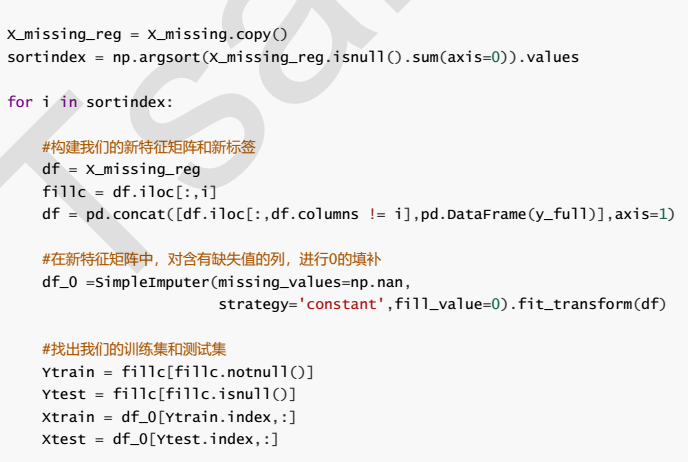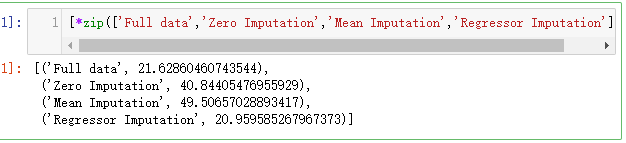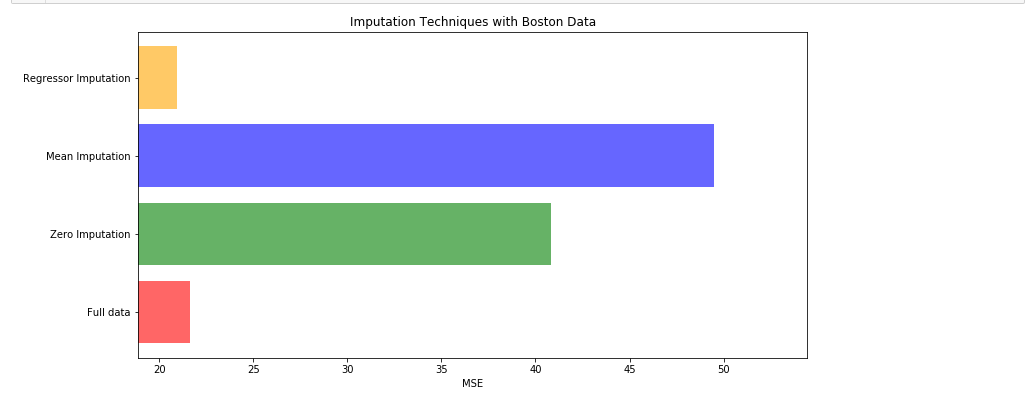• 随机森林填充缺失值 前言： 任何回归都是从特征矩阵中学习，然后求解连续性标签y的值，之所以能实现这个过程，是因为回归算法认为，特征矩阵和标签之前存在着某种关系，实际上特征和标签是可以相互转化的，比如说用...
随机森林填充缺失值
前言：
任何回归都是从特征矩阵中学习，然后求解连续性标签y的值，之所以能实现这个过程，是因为回归算法认为，特征矩阵和标签之前存在着某种关系，实际上特征和标签是可以相互转化的，比如说用地区，环境，附近学校数量预测房价的问题，我们既可以用地区，环境，附近学校数量的数据来预测房价，也可以反过来，用环境，附近学校数量和房价来预测地区，而回归填补缺失值，正式利用了这种情况。
非常使用与一个特征大量数据缺失，其他特征却很完整的情况。
def fill_missing_rf(X,y,to_fill):

"""
使用随机森林填补一个特征的缺失值的函数

参数：
X：要填补的特征矩阵
y：完整的，没有缺失值的标签
to_fill：字符串，要填补的那一列的名称
"""

#构建我们的新特征矩阵和新标签
df = X.copy()
fill = df.loc[:,to_fill]
df = pd.concat([df.loc[:,df.columns != to_fill],pd.DataFrame(y)],axis=1)

# 找出我们的训练集和测试集
Ytrain = fill[fill.notnull()]#特征不缺失的值
Ytest = fill[fill.isnull()]#特征缺失的值
Xtrain = df.iloc[Ytrain.index,:]#特征不缺失的值对应其他n-1个特征+本来的标签
Xtest = df.iloc[Ytest.index,:]#特征缺失的值对应其他n-1个特征+本来的标签

#用随机森林回归来填补缺失值
from sklearn.ensemble import RandomForestRegressor as rfr
rfr = rfr(n_estimators=100)
rfr = rfr.fit(Xtrain, Ytrain)
Ypredict = rfr.predict(Xtest)

return Ypredict

X = data.iloc[:,1:]
y = data["SeriousDlqin2yrs"]#y = data.iloc[:,0]
X.shape#(149391, 10)

#=====[TIME WARNING:1 min]=====#
y_pred = fill_missing_rf(X,y,"MonthlyIncome")

#注意可以通过以下代码检验数据是否数量相同
# y_pred.shape ==  data.loc[data.loc[:,"MonthlyIncome"].isnull(),"MonthlyIncome"].shape

#确认我们的结果合理之后，我们就可以将数据覆盖了
data.loc[data.loc[:,"MonthlyIncome"].isnull(),"MonthlyIncome"] = y_pred

data.info()

问题：对于一个特征缺失，其他特征也有缺失值怎么办？
答案是遍历所有的特征，从缺失最少的特征开始填补（因为填补缺失值最少的特征所需要的准确信息最少）。填补一个特征时，先将其他特征的缺失值用0代题，没完成一次回归预测，就将预测值放到原本的特征矩阵中，再继续填补下一个特征，每一次填补完毕，有缺失值的特征会减少一个，所以每次循环后，需要用0填补的特征就越来越少，当进行到最后一个特征时（这个特征应该时所有特征缺失值最多的），已经没有其他特征需要用0来进行填补，遍历所有的特征后，数据就完整，不再有缺失值。展开全文• 随机森林填充缺失值优点 随机森林填补通过构造多棵决策树对缺失值进行填补，使填补的数据具有随机性和不确定性，更能反映出这些未知数据的真实分布； 由于在构造决策树过程中，每个分支节点选用随机的部分特征而...
为什么要用随机森林对缺失值填补！

缺失值的填充往往会训练的结果，在闲时不妨尝试多种填充然后比较结果选择结果最优的一种；
多学一点又不会死。

随机森林填充缺失值优点

随机森林填补通过构造多棵决策树对缺失值进行填补，使填补的数据具有随机性和不确定性，更能反映出这些未知数据的真实分布；
由于在构造决策树过程中，每个分支节点选用随机的部分特征而不是全部特征，所以能很好的应用到高维数据的填补；
随机森林算法本身就具有很好的分类精度，从而也更进一步确保了得到的填补值的准确性和可靠性。

开始！冲！
import numpy as np
import pandas as pd
from sklearn.impute import SimpleImputer
from sklearn.ensemble import RandomForestRegressor

1.创建数据特征矩阵
data = pd.DataFrame({'id':[1,np.nan,np.nan,np.nan,5,6],'name':[22,np.nan,12,22,377,200],'math':[90,np.nan,99,78,97,np.nan],'english':[89,np.nan,80,94,94,90]})


注意：
在创建数据的时候就把缺失值填到里面了，模拟数据已经存在缺失值

2.创建标签
target = pd.DataFrame({'lable':[1,0,1,1,1,0]})3.根据缺失值的多少对对列名进行升序
sortindex = data.isnull().sum().sort_values().index
sortindex = list(sortindex)
sortindex


[‘name’, ‘english’, ‘math’, ‘id’]

4.开始填充
for i in sortindex:
#构建新特征和新标签
df = data
fillc = df.loc[:,i]
df = pd.concat([df.loc[:,df.columns != i],target],axis=1)
# target 标签

#在新特征中，对有缺失值的列，用0填补 df_0：数组类型
df_0 =SimpleImputer(missing_values=np.nan,strategy='constant',fill_value=0).fit_transform(df)

# 找出训练集和测试集
# 找出当列非空的值
Ytrain = fillc[fillc.notnull()]
# 找出当列空的值
Ytest = fillc[fillc.isnull()]
# 根据非空值找出一整行数组
Xtrain = df_0[Ytrain.index,:]
# 根据空值找出一整行数组
Xtest = df_0[Ytest.index,:]

#⽤随机森林回归来训练预测
rfc = RandomForestRegressor(n_estimators=50)
rfc = rfc.fit(Xtrain, Ytrain)
Ypredict = rfc.predict(Xtest)

#将填补好的特征返回到我们的原始的特征中
data.loc[data.loc[:,i].isnull(),i] = Ypredict

5.填充完成
查看缺失值情况
data.isnull().sum()


id          0
name       0
math       0
english    0
dtype: int64

注意：

1.数据表中必须每一列都得存在缺失值，不然会报错，在实战下可以把存在缺失的字段截下来就可以了，不懂就私聊我
2.n_estimators：树的数量，不是越大就越好，但占用的内存与训练和预测的时间也会相应增长，在可承受的内存/时间内选取尽可能大的n_estimators

希望能够帮助到您，如果可以的话点个赞8，如果有不足的地方请指教，谢谢


展开全文机器学习 数据分析 算法
• 随机森林填补缺失值 这里呢，首先将波士顿数据进行填空，然后在对含有空值的数据进行，0、均值、随机森林回归填充，并比较其好坏，并以图像视之。 首先导入所需要的库 import numpy as np import pandas as pd ...
用随机森林填补缺失值
这里呢，首先将波士顿数据进行填空，然后在对含有空值的数据进行，0、均值、随机森林回归填充，并比较其好坏，并以图像视之。
首先导入所需要的库
import numpy as np
import pandas as pd
import matplotlib.pyplot as plt
from sklearn.impute import SimpleImputer#填补缺失的类
from sklearn.ensemble import RandomForestRegressor

获取数据，并查看数据
dataset = load_boston()#数据
dataset.data.shape#查看数据结构确定样本和标签数量
n_samples = x_full.shape#样本数量
n_features = x_full.shape#标签数量

确定缺失值
#首先确定我们希望放入的缺失值数据的比例，在这里我们假设是50%，那就要有3289个数据缺失

rng = np.random.RandomState(0)
missing_rate = 0.5
n_missing_samples = int(np.floor(n_samples * n_features *missing_rate))


取出将赋值为空值的索引
#np.floor  向下取整
#所有数据要随机遍布在数据集的各行各列当中，而一个确实的数据会需要一盒行索引和一个列索引
#如果能够创造一个数组，就可以利用索引来赋空值

#用0，均值，随机森林来填写这些缺失值，然后查看这些回归结果
missing_features = rng.randint(0,n_features,n_missing_samples)
missing_samples =rng.randint(0,n_samples,n_missing_samples)

#randint(上限，下限，n)  请在下限和上限之间取出n个整数
#我们现在采样了3289个数据，远远超过了我们的样本量，所以可以使用随机抽取的函数randint
将数据赋值为nan
#但是如果我们的数据量小于我们的样本量506，那我们可以采用np.random.choice，choice会随机抽取不重复的随机数
##因此可以帮助我们让数据更加分散，确保数据不会集中在一些行中
x_missing = x_full.copy()
y_missing = y_full.copy()
x_missing[missing_samples,missing_features] = np.nan
x_missing = pd.DataFrame(x_missing)

#转换成DataFrame时为了后面方便操作，索引方便使用均值填补缺失值
#均值
from sklearn.impute import SimpleImputer
imp_mean = SimpleImputer(missing_values=np.nan,strategy='mean')
x_missing_mean = imp_mean.fit_transform(x_missing)
#训练fit+导出predict >>> 特殊的接口fit_transform

pd.DataFrame(x_missing_mean).isnull().sum()#查看缺失值使用 0 填补缺失值
#使用0进行填补
imp_0 = SimpleImputer(missing_values=np.nan,strategy="constant",fill_value=0)#censtant 常数
x_missing_0 = imp_0.fit_transform(x_missing)

pd.DataFrame(x_missing_0).isnull().sum()#查看缺失值#使用随机森林填充
x_missing_reg = x_missing.copy()
sortindex = np.argsort(x_missing_reg.isnull().sum(axis=0)).values
#用argsort 排序可以返回索引位置

for i in sortindex:
#构建我们的新特征矩阵（没有被选中去填充的特征+原始的标签）和新标签（被选中去填充的特征）
df = x_missing_reg
fillc = df.iloc[:,i]
df = pd.concat([df[df != fillc],pd.DataFrame(y_full)])

#在新特征矩阵中，对含有缺失值的列，进行0的填补
df_0 = SimpleImputer(missing_values=np.nan
,strategy = "constant"
,fill_value = 0
).fit_transform(df)

#找出我们的训练集和测试集
y_train = fillc[fillc.notnull()]#是被选中要填充的值，存在的那些值，非空值
y_test = fillc[fillc.isnull()]#是被选中 的要填充给的值，不存在的那些值，是空值
x_train = df_0[y_train.index,:]#在新特征矩阵中，我们需要非空值所对应的的索引
x_test = df_0[y_test.index,:]#空值所对应的记录

#用随机森林回归来填补缺失值
rfc = RandomForestRegressor(n_estimators=100)#实例化
rfc = rfc.fit(x_train,y_train)  #导入训练集去进行训练
Ypredict = rfc.predict(x_test)#用oredicr接口将x_TEST,就是我们要填补空值的这些值

#将填补号的特征返回到我们的原始的特征矩阵中
x_missing_reg.loc[x_missing_reg.iloc[:,i].isnull(),i] = Ypredict对填充好的数据进行建模
from sklearn.model_selection import cross_val_score
x = [x_full,X_missing_mean,x_missing_0,x_missing_reg]

mse = []
std = []
for x in x:
estimator = RandomForestRegressor(random_state=0, n_estimators=100)#实例化
scores = cross_val_score(estimator,x,y_full,scoring='neg_mean_squared_error', cv=5).mean()
mse.append(scores * -1)

[*zip(['Full data','Zero Imputation','Mean Imputation','Regressor Imputation'],mse)]将结果用图的形式展现出来
#画图
x_labels = ['Full data',
'Zero Imputation',
'Mean Imputation',
'Regressor Imputation']
colors = ['r', 'g', 'b', 'orange']

plt.figure(figsize=(12, 6))#画出画布
ax = plt.subplot(111)#添加子图
for i in np.arange(len(mse)):
ax.barh(i, mse[i],color=colors[i], alpha=0.6, align='center')#bar为条形图，barh为横向条形图，alpha表示条的粗度
ax.set_title('Imputation Techniques with Boston Data')
ax.set_xlim(left=np.min(mse) * 0.9,
right=np.max(mse) * 1.1)#设置x轴取值范围
ax.set_yticks(np.arange(len(mse)))
ax.set_xlabel('MSE')
ax.set_yticklabels(x_labels)
plt.show()由此可见随机森林最小，说明效果好，比源数据拟合程度逗号，但是最小不一定是最好的，容易过拟合。


展开全文机器学习 python numpy 过拟合 scheme
• 当缺失值比例小于10%时，可以考虑用随机森林填充缺失值
# 定义随机森林填补函数
def fill_missing_rf(X,y,to_fill):
"""
使用随机森林填补一个特征的缺失值的函数
参数：
X：要填补的特征矩阵
y：完整的，没有缺失值的标签
to_fill：字符串，要填补的那一列的名称
"""
#构建我们的新特征矩阵和新标签
df = X.copy()
fill = df.loc[:,to_fill]
df = pd.concat([df.loc[:,df.columns != to_fill],pd.DataFrame(y)],axis=1)

#找出我们的训练集和测试集
Ytrain = fill[fill.notnull()]
Ytest = fill[fill.isnull()]
Xtrain = df.iloc[Ytrain.index,:]
Xtest = df.iloc[Ytest.index,:]

#用随机森林回归来填补缺失值
from sklearn.ensemble import RandomForestRegressor as rfr
rfr = rfr(n_estimators = 100)
rfr = rfr.fit(Xtrain, Ytrain)
Ypredict = rfr.predict(Xtest)

return Ypredict



展开全文• UCI机器学习练习数据集网站：http://archive.ics.uci.edu/ml/datasets/Skin+Segmentation竞赛中备青睐的算法：随机森林、梯度提升树（GBDT）、XGBoost等集成树... 随机森林分类器/随机森林回归器【bagging使用的两个...
• RF中有相应的缺失值处理方法，本次记录其两种缺失值处理技巧 暴力填补 Python中的na.roughfix包提供简单的缺失值填补策略： 对于训练集中处于同一个类别下的数据，如果是类别变量缺失，则用众数补全，如果是连续...
• 在scikit-learn中，有一个专门填充的类，专门将均值，中值，或其他数值填充，比如还有0值填充随机森林回归填充，来分别验证拟合状况，来找出此数据集最佳的缺失值填补方式。 假如我们还是使用回归数据来做测试，...机器学习
• 缺失值的分类 按照数据缺失机制可分为： 可忽略的缺失 完全随机缺失，所缺失的数据发生的概率既与已观察到的数据无关，也与未观察到的数据无关 随机缺失，假设缺失数据发生的概率与所观察到的变量是有关的，而与未...
• 随机森林 from sklearn import ensemble from sklearn.preprocessing import LabelEncoder def set_missing(df,estimate_list,miss_col): """df要处理的数据帧，estimate_list用来估计缺失值的字段列表,miss_col...